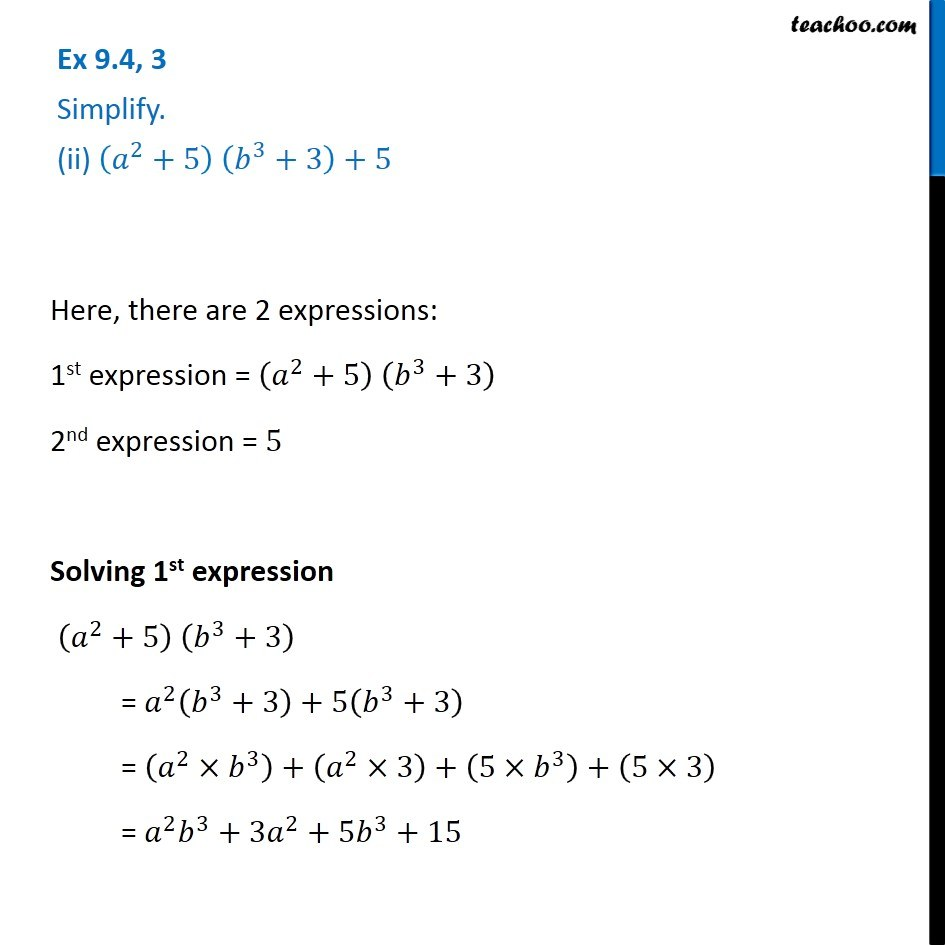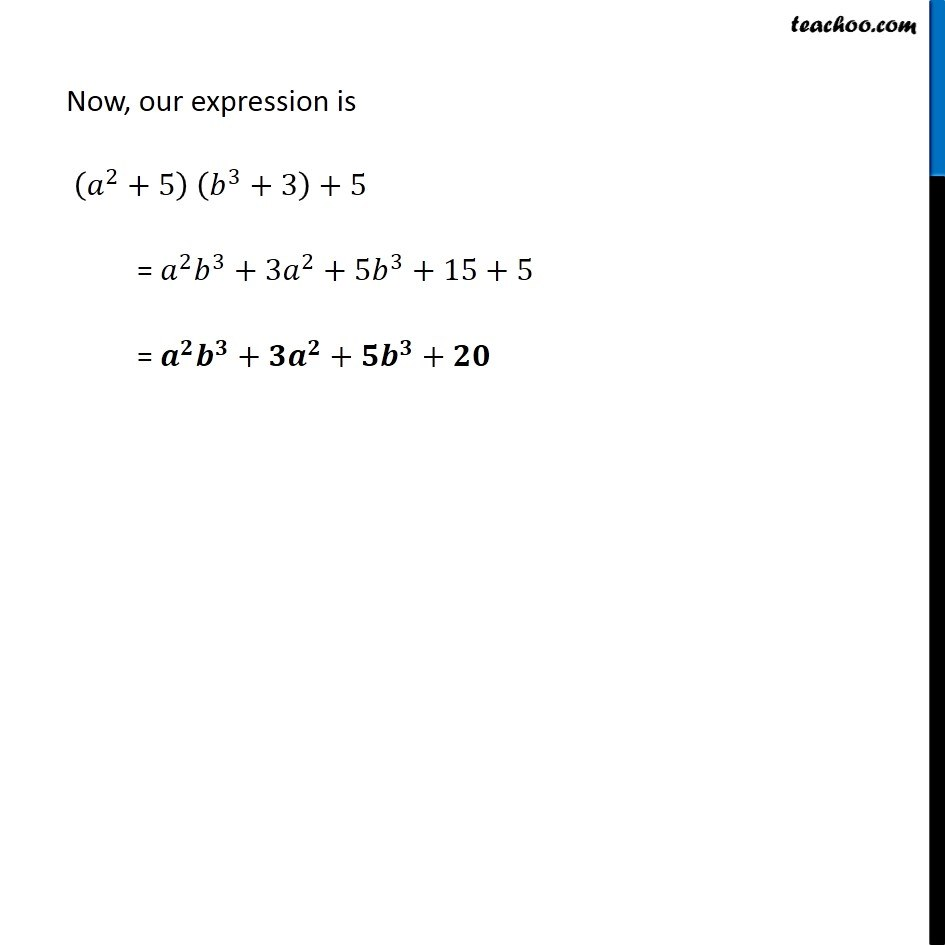Subscribe to our Youtube Channel - https://you.tube/teachoo

1. Chapter 9 Class 8 Algebraic Expressions and Identities
2. Concept wise
3. Multiplication of Polynoimals by Polynomials

Transcript

Ex 9.4, 3 Simplify. (ii) (𝑎^2+5) (𝑏^3+3)+5 Here, there are 2 expressions: 1st expression = (𝑎^2+5) (𝑏^3+3) 2nd expression = 5 Solving 1st expression (𝑎^2+5) (𝑏^3+3) = 𝑎^2 (𝑏^3+3)+5(𝑏^3+3) = (𝑎^2×𝑏^3 )+(𝑎^2×3)+(5×𝑏^3 )+(5×3) = 𝑎^2 𝑏^3+3𝑎^2+5𝑏^3+15 Now, our expression is (𝑎^2+5) (𝑏^3+3)+5 = 𝑎^2 𝑏^3+3𝑎^2+5𝑏^3+15+5 = 𝒂^𝟐 𝒃^𝟑+𝟑𝒂^𝟐+𝟓𝒃^𝟑+𝟐𝟎

Multiplication of Polynoimals by Polynomials

About the AuthorDavneet Singh
Davneet Singh is a graduate from Indian Institute of Technology, Kanpur. He has been teaching from the past 9 years. He provides courses for Maths and Science at Teachoo.